Successfully reported this slideshow.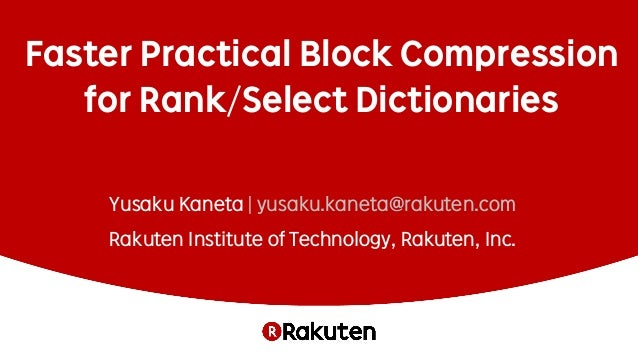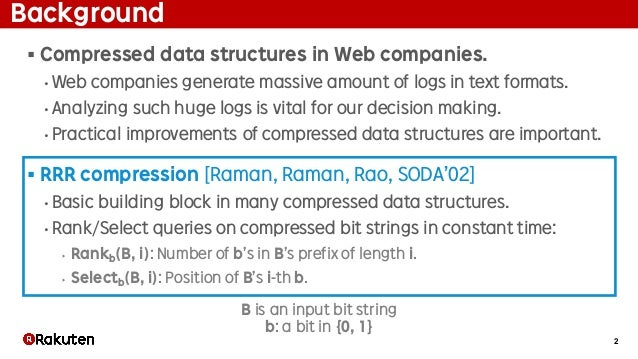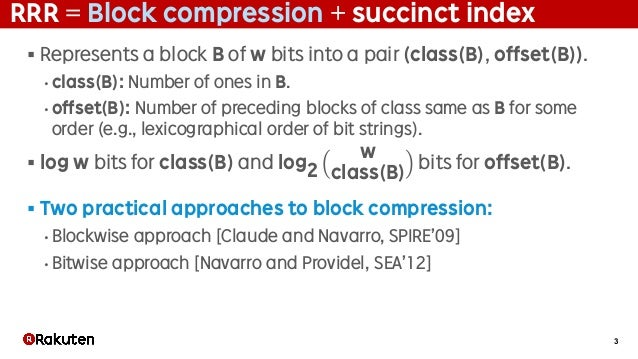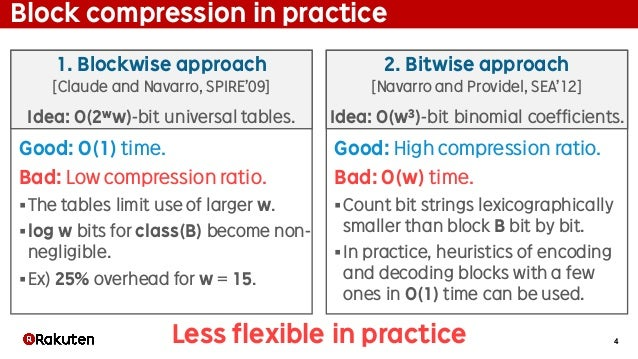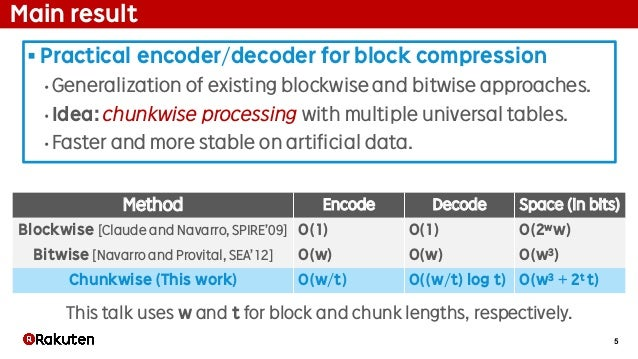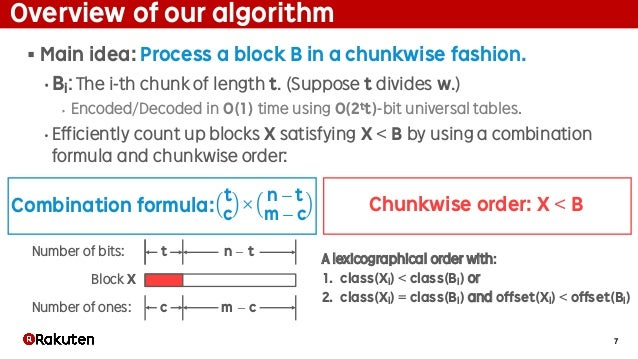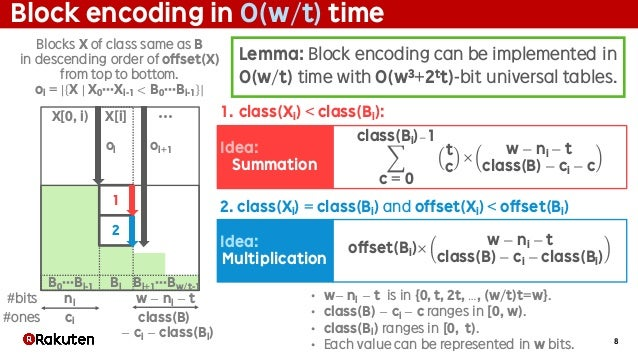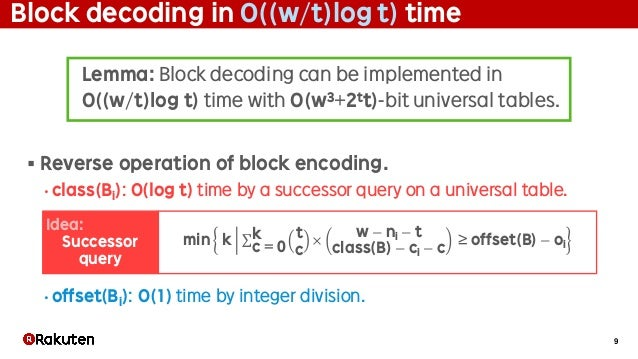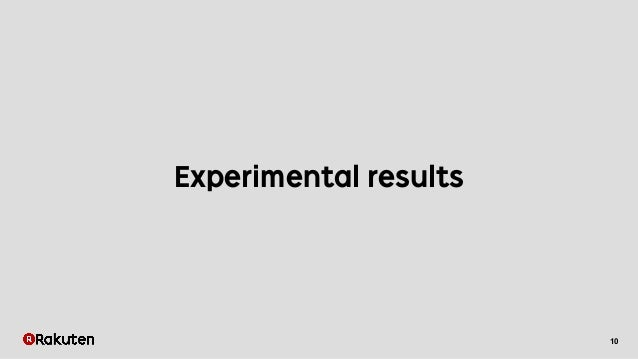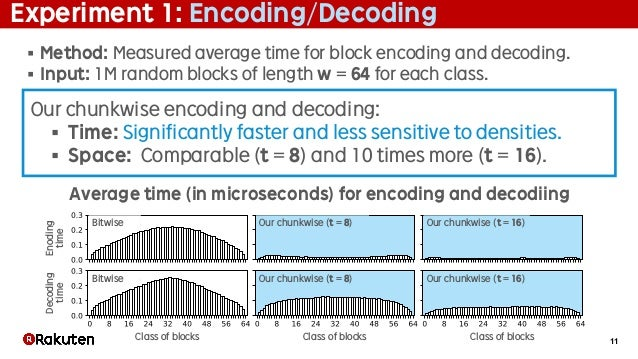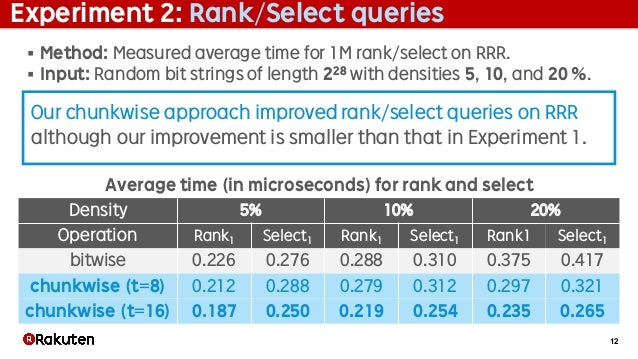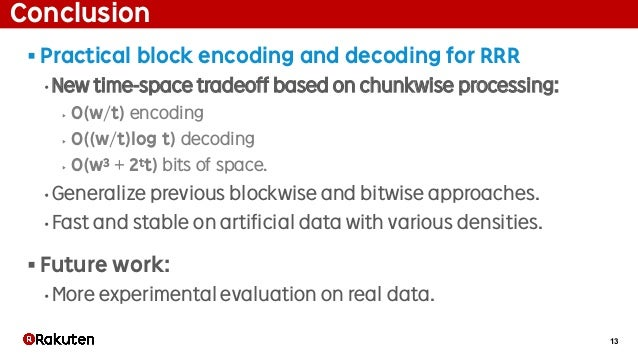Upcoming SlideShare
×

# Faster Practical Block Compression for Rank/Select Dictionaries

We present faster practical encoding and decoding procedures for block compression. Such encoding and decoding procedures are important to efficiently support rank/select queries on compressed bit vectors. This paper was presented at the 24th International Symposium on String Processing and Information Retrieval (SPIRE 2017) in Palermo, Italy.

• Full Name
Comment goes here.

Are you sure you want to Yes No• Be the first to comment

### Faster Practical Block Compression for Rank/Select Dictionaries

1. 1. Faster Practical Block Compression for Rank/Select Dictionaries Yusaku Kaneta | yusaku.kaneta@rakuten.com Rakuten Institute of Technology, Rakuten, Inc.
2. 2. 2 Background § Compressed data structures in Web companies. • Web companies generate massive amount of logs in text formats. • Analyzing such huge logs is vital for our decision making. • Practical improvements of compressed data structures are important. § RRR compression [Raman, Raman, Rao, SODA’02] • Basic building block in many compressed data structures. • Rank/Select queries on compressed bit strings in constant time: ‣ Rankb(B, i): Number of b’s in B’s prefix of length i. ‣ Selectb(B, i): Position of B’s i-th b. B is an input bit string b: a bit in {0, 1}
3. 3. 3 RRR = Block compression + succinct index § Represents a block B of w bits into a pair (class(B), offset(B)). • class(B): Number of ones in B. • offset(B): Number of preceding blocks of class same as B for some order (e.g., lexicographical order of bit strings). § log w bits for class(B) and log2 w class(B) bits for offset(B). § Two practical approaches to block compression: • Blockwise approach [Claude and Navarro, SPIRE’09] • Bitwise approach [Navarro and Providel, SEA’12]
4. 4. 4 Block compression in practice Good: O(1) time. Bad: Low compression ratio. §The tables limit use of larger w. §log w bits for class(B) become non- negligible. §Ex) 25% overhead for w = 15. 1. Blockwise approach [Claude and Navarro, SPIRE’09] 2. Bitwise approach [Navarro and Providel, SEA’12] Idea: O(2ww)-bit universal tables. Idea: O(w3)-bit binomial coefficients. Good: High compression ratio. Bad: O(w) time. §Count bit strings lexicographically smaller than block B bit by bit. §In practice, heuristics of encoding and decoding blocks with a few ones in O(1) time can be used. Less flexible in practice
5. 5. 5 Main result § Practical encoder/decoder for block compression • Generalization of existing blockwise and bitwise approaches. • Idea: chunkwise processing with multiple universal tables. • Faster and more stable on artificial data. Method Encode Decode Space (in bits) Blockwise [Claude and Navarro, SPIRE’09] O(1) O(1) O(2ww) Bitwise [Navarro and Provital, SEA’12] O(w) O(w) O(w3) Chunkwise (This work) O(w/t) O((w/t) log t) O(w3 + 2t t) This talk uses w and t for block and chunk lengths, respectively.
6. 6. 6 Our algorithm
7. 7. 7 Overview of our algorithm § Main idea: Process a block B in a chunkwise fashion. • Bi: The i-th chunk of length t. (Suppose t divides w.) ‣ Encoded/Decoded in O(1) time using O(2tt)-bit universal tables. • Efficiently count up blocks X satisfying X < B by using a combination formula and chunkwise order: A lexicographical order with: 1. class(Xi) < class(Bi) or 2. class(Xi) = class(Bi) and offset(Xi) < offset(Bi) t c × n − t m − c c m − c n − tt Number of ones: Number of bits: Block X Combination formula: Chunkwise order: X < B
8. 8. 8 Block encoding in O(w/t) time Lemma: Block encoding can be implemented in O(w/t) time with O(w3+2tt)-bit universal tables. ` 1 oi+1 B0···Bi-1 oi 2 X[0, i) X[i] ••• Blocks X of class same as B in descending order of offset(X) from top to bottom. oi = X X0···Xi-1 < B0···Bi-1 ci ni class(B) − ci − class(Bi) w − ni − t#bits #ones Bi Bi+1···Bw/t-1 • w− ni − t is in {0, t, 2t, …, (w/t)t=w}. • class(B) − ci − c ranges in [0, w). • class(Bi) ranges in [0, t). • Each value can be represented in w bits. 2. class(Xi) = class(Bi) and offset(Xi) < offset(Bi) Idea: Multiplication offset(Bi)× w − ni − t class(B) − ci − class(Bi) 1. class(Xi) < class(Bi): Idea: Summation % t c × w − ni − t class(B) − ci − c class(Bi)&1 c = 0
9. 9. 9 Block decoding in O((w/t)log t) time § Reverse operation of block encoding. • class(Bi): O(log t) time by a successor query on a universal table. • offset(Bi): O(1) time by integer division. min k ∑ t c × w − ni − t class(B) − ci − c ≥ offset(B) − oi k c = 0 Lemma: Block decoding can be implemented in O((w/t)log t) time with O(w3+2tt)-bit universal tables. Idea: Successor query
10. 10. 10 Experimental results
11. 11. 11 Experiment 1: Encoding/Decoding § Method: Measured average time for block encoding and decoding. § Input: 1M random blocks of length w = 64 for each class. Our chunkwise encoding and decoding: § Time: Significantly faster and less sensitive to densities. § Space: Comparable (t = 8) and 10 times more (t = 16). Average time (in microseconds) for encoding and decodiing Bitwise Bitwise Our chunkwise (t = 8) Our chunkwise (t = 8) Our chunkwise (t = 16) Our chunkwise (t = 16) Class of blocks Class of blocks Class of blocks Decoding time Enoding time
12. 12. 12 Experiment 2: Rank/Select queries § Method: Measured average time for 1M rank/select on RRR. § Input: Random bit strings of length 228 with densities 5, 10, and 20 %. Density 5% 10% 20% Operation Rank1 Select1 Rank1 Select1 Rank1 Select1 bitwise 0.226 0.276 0.288 0.310 0.375 0.417 chunkwise (t=8) 0.212 0.288 0.279 0.312 0.297 0.321 chunkwise (t=16) 0.187 0.250 0.219 0.254 0.235 0.265 Average time (in microseconds) for rank and select Our chunkwise approach improved rank/select queries on RRR although our improvement is smaller than that in Experiment 1.
13. 13. 13 Conclusion § Practical block encoding and decoding for RRR • New time-space tradeoff based on chunkwise processing: ‣ O(w/t) encoding ‣ O((w/t)log t) decoding ‣ O(w3 + 2tt) bits of space. • Generalize previous blockwise and bitwise approaches. • Fast and stable on artificial data with various densities. § Future work: • More experimental evaluation on real data.
14. 14. THANK YOU

### Be the first to comment

•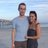#### georgeericksonjr

Nov. 1, 2018
•#### NazifSaiyed

Mar. 18, 2019

Total views

11,072

On Slideshare

0

From embeds

0

Number of embeds

10,340

4

Shares

0